Home > INT1 > Chapter Ch7 > Lesson 7.1.5 > Problem7-63

7-63.

Solve for the indicated variable by rewriting each equation. Homework Help ✎

1. V = LWH (for W)

2. y =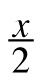+ 3 (for x)

3.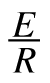= I (for R)

4. 2x +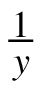= 3 (for y)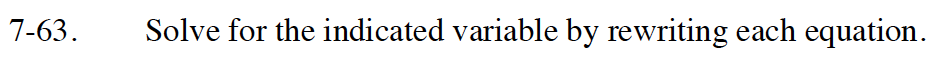Read the Math Notes box for section 3.3.1 to review properties for solving equations.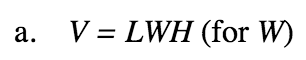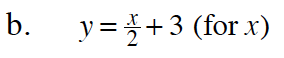The equation contains multiplication. To undo the multiplication divide both sides by L and H.

When solving an equation, use the Addition or Subtraction Property of Equality first to isolate the term with the variable. In this case you will be adding the opposite of 3. Then use the Multiplicative Property of Equality.

x = 2(y − 3) = 2y − 6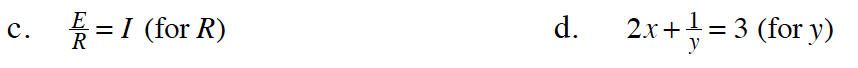Use the Multiplicative Property of Equality and multiply both sides of the equation by R.

Add −2x to both sides of the equation.

Multiply both sides of the equation by y.

Divide both sides of the equation by (3 − 2x).

$y=\frac{1}{\left(-2x+3\right)}$